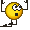# Calculating frequency of sin(x)

• B
Greetings,

I'm confused about calculating frequency of sin(x) function.

Applying basic equations I get this:

T= 2pi

f = 1/T = 1/(2pi) = 1/6.28 = 0.15923 Hz

I know it's wrong, but what am I missing?

Also, how can the period be in radians, I always thought that T should be in seconds.

Related Other Physics Topics News on Phys.org
DrClaude
Mentor
##\sin(x)## is not a function of time, at least, not explicitly. So let's make it explicit:
$$\sin(\omega t)$$
or
$$\sin(2 \pi \nu t)$$
where ##\omega## is the angular frequency, expressed in radians per second (often simply written as 1/s, without the radian part) and ##\nu = \omega / 2 \pi## is the frequency, expressed in Hz, you are after.

•sophiecentaur
so for sin(x), ω = 1

then my calculation f = ω/2π = 1/6.28 = 0.15923 Hz should be correct?

what am I missing?
The whole meaning and purpose of calculating a frequency. Why are you using ##\sin(x)##? What is ##x##? Besides, period in physics is something a little different than in pure maths.

This discuss maths, not physics as far as I can see. Period in maths is something different than in physics.

ω should be 1 so the frequeny f = ω/2π = 1/6.28 = 0.15923 Hz

Is this correct?

Yes (if ##\omega## is expressed in ##\frac{1}{s}##).

Last edited:
ZapperZ
Staff Emeritus
Thus is mathematically wrong. The argument for any trig function must be dimensionless. You cannot use sin(t) if t is time. It must be of the form sin(ωt).

Zz.

•Lord Jestocost
sophiecentaur
Gold Member

ω should be 1 so the frequeny f = ω/2π = 1/6.28 = 0.15923 Hz

Is this correct?
I think you would find this a lot easier if you try to ignore your 'private' way of looking at the topic and just followed the mainstream. You could "how about" a lot if ideas but you will make faster progress if you try to go along with the usual treatment - it was developed for a good reason and yields some useful understanding.

PS this is not just a "Do it this way and stop thinking" message. People can often feel victimised when they try things out on PF but people have some pretty fair ideas on this site.

•BvU
FactChecker
Gold Member
Thus is mathematically wrong. The argument for any trig function must be dimensionless. You cannot use sin(t) if t is time. It must be of the form sin(ωt).
It is common to express any function of time as f(t). The sin function is as good as any function. IMO, there is no reason to insist that it be treated differently. In that context, the period is 0.159.

••Merlin3189 and DrClaude
sophiecentaur
Gold Member
The sin function is as good as any function.
True but the units are not intuitive and how do you deal conveniently with a mixture of several frequencies at once??. It's hardly surprising that 'everyone' uses ωt, is it?
Using Sin(t) is standing up in a hammock - I think we can all agree - if pushed.
It is common to express any function of time as f(t)
The function Exp(-t) is another one which, refusing to include a scale constant inside the brackets really does make life difficult and for no good reason. Where would we be without our e and our ω (unless we were pure mathematicians, of course).

•gmax137 and FactChecker
George Jones
Staff Emeritus
Gold Member
It is common to express any function of time as f(t). The sin function is as good as any function. IMO, there is no reason to insist that it be treated differently. In that context, the period is 0.159.
It is certainly true the sine function is periodic in its argument, whatever the interpretation of its argument. It is very unusual in physics, however, for the argument of sine to have physical units. For example, if ##t## has the units of seconds, what are the units of
$$\sin t = t - \frac{t^3}{3!} + \frac{t^5}{5!} - ... ?$$

If ##t## has units of seconds, then, on the right, the physical units of the first term are ##s##, the units of the second term are ##s^3##, etc. How do we sum these terms to get a physically sensible answer? If ##t## has no physical units, then the sum makes physical sense.

•gmax137, FactChecker, Nugatory and 3 others
standing up in a hammock
Thanks, sophie, I never heard that beforesophiecentaur
•